# Hypothesis Testing | All a beginner needs to know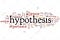Source

Hypothesis in terms of statistics is an assumption for an event, a proportion based on reasoning. Hypothesis testing is a statistical method used to make decisions using experimental data. Basically, we are assuming a result using some parameters for a problem statement.

Didn’t get it? OK…

Suppose you run a Pharmaceutical company, and you have launched a drug which is in the market for quite a long time. Now you want to know how many percentages of the Indian population use this drug when they have related diseases to forecast the drug's future production. …

# Probability Distributions in Data Science and Machine Learning | Part 2

Note: This blog is a continuum of “Probability Distributions in Data Science and Machine Learning | Part 1”. In case you haven’t read it yet, here is the link.

Here I am going to discuss various types of continuous probability distributions and their application in machine learning.

# Continuous Probability Distributions:

Some of the standard continuous probability distributions are

1. Normal/Gaussian
2. Student’s t-distribution
3. Exponential
4. log-normal
5. Power law and Pareto distribution

# Normal/Gaussian Distribution:

The normal distribution is the backbone of statistics and data science. Many machine learning models work well with data that follow a normal distribution. Such as;

1. Gaussian Naive Bayes Classifier
2. Logistic, Linear Regression, and least…

# Probability Distributions in Data Science and Machine Learning | Part 1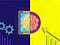analyticsindiamag.com

For a data scientist aspirant, Statistics is a must-learn thing. It can process complex and challenging problems in the real world so that Data Scientists can mine useful trends, changes, and data behavior to fit into the appropriate model, yielding the best results. Every time we get a new dataset, we must understand the data pattern and the underlying probability distribution for further optimization and treatment during the Exploratory Data Analysis(EDA). During EDA, we try to find out the behavior of data using different probability distributions. …

# Central Limit Theorem and Machine Learning | Part-2

This blog is a continuation of “Central Limit Theorem and Machine Learning”. Please visit the part-1 for prior knowledge about the topic. The link is here.

As we discussed earlier, we can’t simply assume a sample’s mean parameter output as our whole population parameter output though their values are close. We need to validate this uncertainty using a Confidence Interval methodology.

# What is Confidence Interval?

In statistics, a confidence interval refers to the probability that a population parameter will fall between a set of values for a certain proportion of times. Confidence intervals measure the degree of uncertainty or certainty in a sampling method…

# Central Limit Theorem and Machine Learning | Part-1

Note: Here I will try to cover the idea of the Central Limit Theorem, and it’s significance in statistical analysis, and how it is useful in Machine Learning. In case you haven’t checked, please find the link for normal distribution blog here.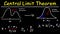Source: Google

Suppose we want to study the average age of the whole population of India. As the popullation of India is very high, it will be a tedious job to get everyone’s age data and will take lot of time for the survey. So instead of doing that we can collect samples from different parts of India and try…

# Normal Distribution and Machine Learning

Normal Distribution is an important concept in statistics and the backbone of Machine Learning. A Data Scientist needs to know about Normal Distribution when they work with Linear Models(perform well if the data is normally distributed), Central Limit Theorem, and exploratory data analysis.

As discovered by Carl Friedrich Gauss, Normal Distribution/Gaussian Distribution is a continuous probability distribution. It has a bell-shaped curve that is symmetrical from the mean point to both halves of the curve.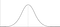Source: Google

## Mathematical Definition:

A continuous random variable “x” is said to follow a normal distribution with parameter μ(mean) and σ(standard deviation), if it’s probability density function is given…

# Correlation and Machine Learning

In a statistical study, which may be scientific, economic, social studies, or machine learning, sometimes we come across many problems involving the use of two or more variables. Such as:

1. Income and Expenditure of an individual
2. Demand and price of a commodity

The data generated by the two variables(x, y) are called the bivariate Data, and this bivariate analysis is critical to find a relationship between them.

# What is Correlation?

The mutual relationship, covariation, or association between two or more variables is called Correlation. …

# A Self Case Study on Quora Insincere Question Classification

Note: Here, I will try to explain how I approach the problem statement starting from exploratory data analysis to model building using deep learning approach end to end. Please do enjoy and give it a clap!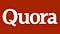Source : Google

Quora is an American question-and-answer website that provides a platform to ask questions and connect with people who contribute unique insight and share quality thoughts via their answers to its users. It empowers people to learn from each other.

Handling harmful content is a big set of problems for websites nowadays. Quora also face the same toxicity via insincere questions posted on its website…## Abhishek Barai

Data Scientist | NLP Engineer | Quantitative Researcher | Blogger